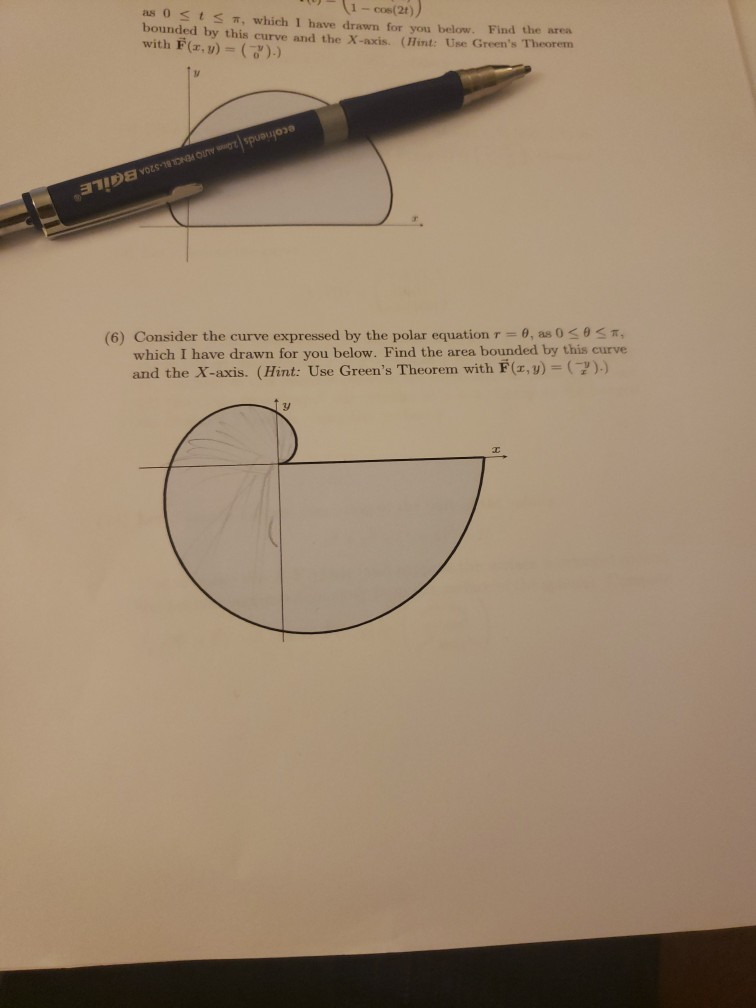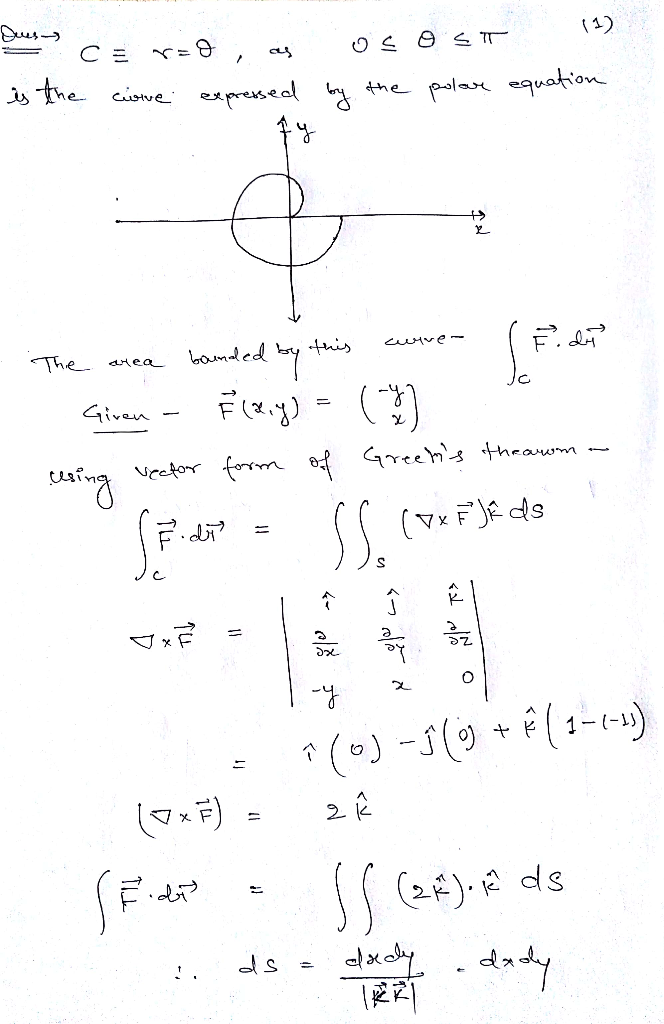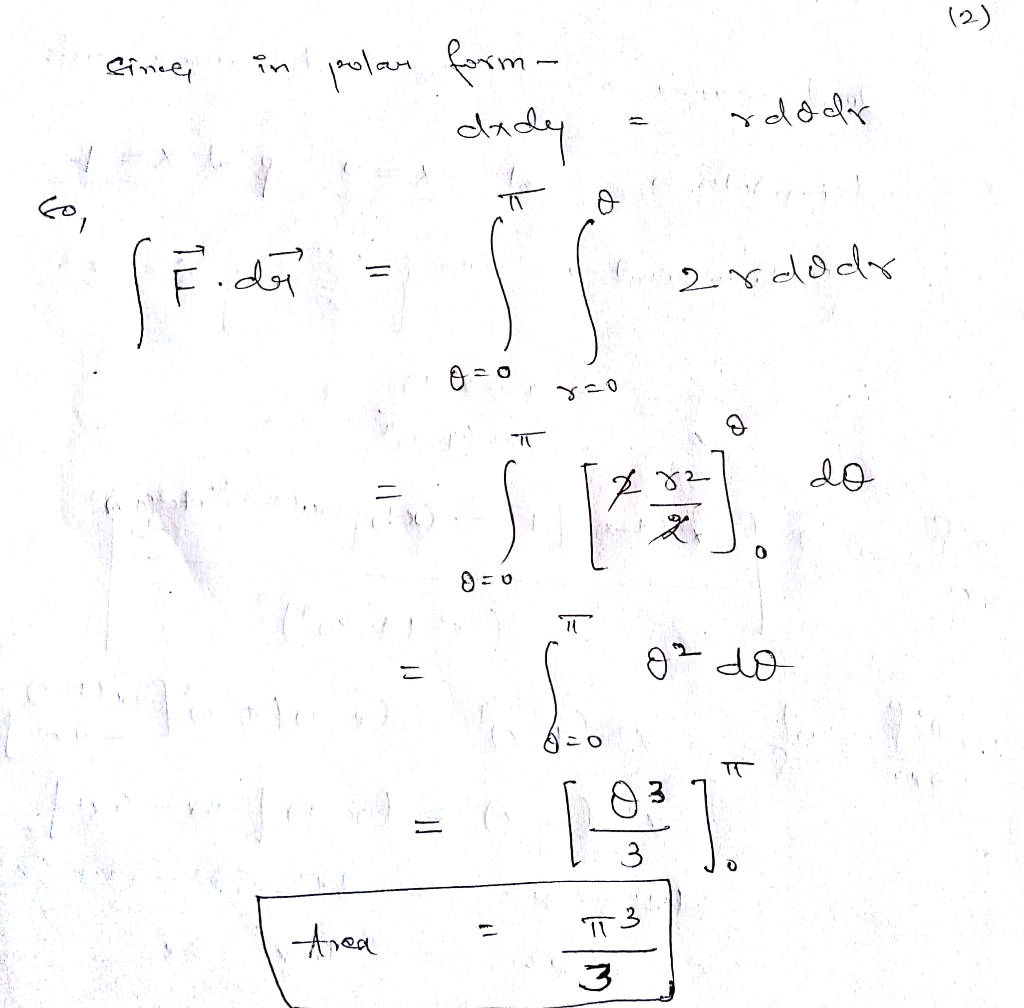Homework Help Question & Answers

1-Cos(24) as O t 7, which I have drawn for you below. Find the area bounded by this curve and the...1-Cos(24) as O t 7, which I have drawn for you below. Find the area bounded by this curve and the X-axis. (Hint: Use Green's Theorem with F(r, y) ()) m, which I have drawn for you below. Find the area bounded by this curve and the X-axis. (Hint: Use Green's Theorem with F(x,)-().) (6) Consider the curve expressed by the polar equation T-0, as 0Add Answer of: 1-Cos(24) as O t 7, which I have drawn for you below. Find the area bounded by this curve and the...
More Homework Help Questions Additional questions in this topic.

• cos(tan + cot) = csc only simplify one side to equal csc so far I got this far: [((cos)(sin))/(cos)] + [((cos)(cos))/(sin)] = csc I don't know what to do next

Need Online Homework Help?

Get FREE EXPERT Answers
WITHIN MINUTES
Related Questions